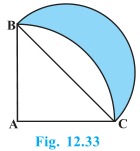# Calculate the area of the designed region in Fig. common between the two quadrants of circles of radius 8 cm each.Solution

AB = BC = CD = AD = 8 cm

Area of ΔABC = Area of ΔADC

Area of triangle=(½)×8×8 = 32 cm2

= 352/7 cm2

= (352/7 -32)+(352/7- 32) cm2

= 2×(352/7-32) cm2

= 256/7 cm2

= 36.57cm2